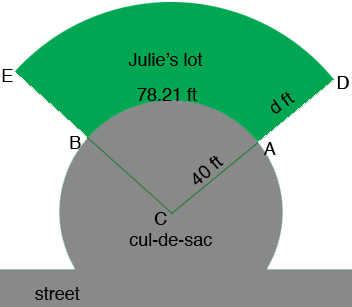SEARCH HOMEMath Central Quandaries & QueriesQuestion from ana, a student: Julie lives on a cul-de-sac and wants to know if the city calculated the area of her plot of land correctly (see diagram below). We can assume the cul-de-sac is a circle and it has radius 40 feet.The boundary of the lot along the cul-de-sac (the arc from A to B) is 78.21 feet. The city found the area to be 7012.5 square feet–are they correct?Hi Ana,

Without the diagram this is difficult. I can guess what the diagram looks like but I think there is a piece of information missing.

Penny

Ana wrote back.

With the diagram may you help me please?

You still didn't send a diagram so the best I can do is guess. I think the diagram looks something like this.I want to find the area of the sector $ABC$ and to do so I am going to use the symmetry of the circle. The area of the sector $ABC$ is a fraction of the area of the circle of radius 40 ft. The length of the arc $AB$ is a fraction of the circumference of the circle of radius 40 ft. By the symmetry of the circle these two fractions are the same. Thus

$\frac{\mbox{area of the arc ABC}}{\pi \; 40^2} = \frac{78.21}{2 \pi \; 40}$

and hence

$\mbox{area of the arc ABC} = \frac{78.21}{2 \pi \; 40} \times \pi \; 40^2 = 156.24 \mbox{ square feet.}$

Do you know the depth of Julie's lot, the distance $d$ in my diagram? If so you can use the procedure I used above to find the area of the sector $DEC,$ and then the area of Julie's lot is the difference in the areas of the two sectors.

I hope this helps,

PennyMath Central is supported by the University of Regina and the Imperial Oil Foundation.